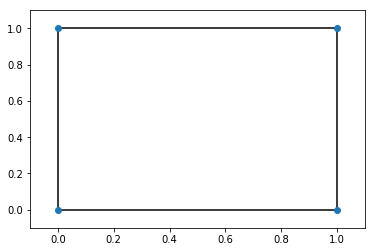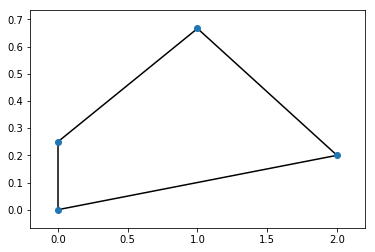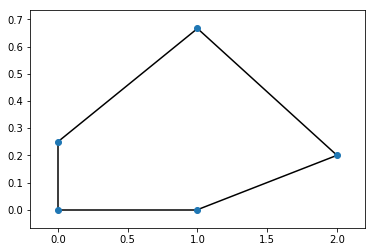# Best response polytopes - solutions¶

1. Define a polytope as a convex hull

2. Define a polytope as an intersection of halfspaces

3. For the following set of vertices draw the corresponding polytope and obtain their definition as an intersection of halfspaces:

A. V = $\{(0, 0), (0, 1), (1, 0), (1, 1)\}$

In :
%matplotlib inline

import matplotlib.pyplot as plt
import numpy as np
import scipy.spatial

V = [np.array([0, 0]), np.array([0, 1]), np.array([1, 0]), np.array([1, 1])]
P = scipy.spatial.ConvexHull(V)
scipy.spatial.convex_hull_plot_2d(P);The definition as an intersection of halfspaces:

\begin{align} - x_1 & \leq 0\\ -x_2 & \leq 0\\ x_1 & \leq 1\\ x_2 & \leq 1 \end{align}

B. V = $\{(0, 0), (0, 1/4), (1, 2/3), (2, 1/5)\}$

In :
V = [np.array([0, 0]), np.array([0, 1 / 4]), np.array([1, 2  / 3]), np.array([2, 1 / 5])]
P = scipy.spatial.ConvexHull(V)
scipy.spatial.convex_hull_plot_2d(P);Let us first find the equations for each boundary:

• Between $(0, 0)$ and $(0, 1/4)$: $x_1=0$
• Between $(0, 1/4)$ and $(1, 2/3)$: $x_2=(5/12)x_1+1/4$
• Between $(1, 2/3)$ and $(2, 1/5)$: $x_2=(-7/15)x_1+17/15$
• Between $(2, 1/5)$ and $(0, 0)$: $x_2=(1/10)x_1$

The definition as an intersection of halfspaces (obtained by identifying the lines corresponding to each boundary of the polytope):

\begin{align} -x_1 & \leq 0\\ 12x_2 - 5x_1 & \leq 3\\ 15x_2 + 7x_1 & \leq 17\\ x_1 -10x_2 & \leq 0 \end{align}

C. V = $\{(0, 0), (0, 1/4), (1, 2/3), (2, 1/5), (1, 0)\}$

In :
V = [np.array([0, 0]), np.array([0, 1 / 4]), np.array([1, 2  / 3]), np.array([2, 1 / 5]), np.array([1, 0])]
P = scipy.spatial.ConvexHull(V)
scipy.spatial.convex_hull_plot_2d(P);Let us first find the equations for each boundary:

• Between $(0, 0)$ and $(0, 1/4)$: $x_1=0$
• Between $(0, 1/4)$ and $(1, 2/3)$: $x_2=(5/12)x_1+1/4$
• Between $(1, 2/3)$ and $(2, 1/5)$: $x_2=(-7/15)x_1+17/15$
• Between $(2, 1/5)$ and $(1, 0)$: $x_2=(1/5)x_1-1/5$
• Between $(1, 0)$ and $(0, 0)$: $x_2=0$

The definition as an intersection of halfspaces (obtained by identifying the lines corresponding to each boundary of the polytope):

\begin{align} -x_1 & \leq 0\\ 12x_2 - 5x_1 & \leq 3\\ 15x_2 + 7x_1 & \leq 17\\ x_1 - 5 x_2 & \leq 1\\ -x_2 & \leq 0 \end{align}

4. Define the best response polytopes.

5. For the following games, obtain the best response polytopes, label the vertices and identify all Nash equilibria:

1. $A = \begin{pmatrix} 3 & -1\\ 2 & 7\end{pmatrix} \qquad B = \begin{pmatrix} -3 & 1\\ 1 & -6\end{pmatrix}$

First we need nonegative valued matrices:

$$A \to A + 2 = \begin{pmatrix} 5 & 1\\ 4 & 9\end{pmatrix} \qquad B \to B + 7 = \begin{pmatrix} 4 & 8\\ 8 & 1\end{pmatrix}$$

The inequalities of $\mathcal{P}$ are then given by:

\begin{align} -x_1 & \leq 0\\ -x_2 & \leq 0\\ 4x_1 + 8x_2 & \leq 1\\ 8x_1 + 1x_2 & \leq 1\ \end{align}

which corresponds to:

\begin{align} x_1 & \geq 0\\ x_2 & \geq 0\\ x_2 & \leq 1/8 - (1/2)x_1\\ x_2 & \leq 1 - 8x_1 \end{align}

The vertices are given by:

$$V=\{(0, 0), (0, 1/8), (1/8, 0), (7/60, 1/15)\}$$

The labels of our vertices (using the ordering of the inequalities):

• $(0, 0)$: $\{0, 1\}$
• $(0, 1/8)$: $\{0, 2\}$
• $(1/8, 0)$: $\{1, 3\}$
• $(7/60, 1/15)$: $\{2, 3\}$

The inequalities of $\mathcal{Q}$ are given by:

\begin{align} 5x_1 + x_2 & \leq 1\\ 4x_1 + 9x_2 & \leq 1\\ -x_1 & \leq 0\\ -x_2 & \leq 0 \end{align}

which corresponds to:

\begin{align} x_2 & \leq 1 - 5x_1\\ x_2 & \leq 1/9 - (4/9)x_1\\ x_1 & \geq 0\\ x_2 & \geq 0 \end{align}

The vertices are given by:

$$V=\{(0, 0), (0, 1/9), (1/5, 0), (8/41, 1/41)\}$$

The labels of our vertices (using the ordering of the inequalities):

• $(0, 0)$: $\{2, 3\}$
• $(0, 1/9)$: $\{1, 2\}$
• $(1/5, 0)$: $\{0, 3\}$
• $(8/41, 1/41)$: $\{0, 1\}$

The only fully labeled vertex pair is given by:

$$((7/60, 1/15), (8/41, 1/41))$$

which gives the normalised Nash equilibrium:

$$((7/11, 4/11), (8/9, 1/9))$$

Here is some code to verify this numerically:

In :
import nashpy as nash
# Obtaining the row player best response polytope vertices
B = np.array([[4, 8], [8, 1]])
halfspaces = nash.polytope.build_halfspaces(B.transpose())
for v, l in nash.polytope.non_trivial_vertices(halfspaces):
print(v)

[0.125 0.   ]
[0.    0.125]
[0.11666667 0.06666667]

In :
# Obtaining the column player best response polytope vertices
A = np.array([[5, 1], [4, 9]])
halfspaces = nash.polytope.build_halfspaces(A)
for v, l in nash.polytope.non_trivial_vertices(halfspaces):
print(v)

[6.93889390e-18 1.11111111e-01]
[0.19512195 0.02439024]
[ 2.0000000e-01 -6.9388939e-18]

In :
# Verifying the Nash equilibria:
A = A - 2  # Scaling back down (note this makes no difference)
B = B - 7
game = nash.Game(A, B)
list(game.vertex_enumeration()), (7/11, 4/11), (8/9, 1/9)

Out:
([(array([0.63636364, 0.36363636]), array([0.88888889, 0.11111111]))],
(0.6363636363636364, 0.36363636363636365),
(0.8888888888888888, 0.1111111111111111))

2. $A = \begin{pmatrix} 2 & -1\\ 1 & 3\end{pmatrix} \qquad B = \begin{pmatrix} -2 & 2\\ 1 & -2\end{pmatrix}$

First we need nonegative valued matrices:

$$A \to A + 2 = \begin{pmatrix} 4 & 1\\ 3 & 5\end{pmatrix} \qquad B \to B + 3 = \begin{pmatrix} 1 & 5\\ 4 & 1\end{pmatrix}$$

The inequalities of $\mathcal{P}$ are then given by:

\begin{align} -x_1 & \leq 0\\ -x_2 & \leq 0\\ x_1 + 4x_2 & \leq 1\\ 5x_1 + x_2 & \leq 1\ \end{align}

which corresponds to:

\begin{align} x_1 & \geq 0\\ x_2 & \geq 0\\ x_2 & \leq 1/4 - (1/4)x_1\\ x_2 & \leq 1 - 5x_1 \end{align}

The vertices are given by:

$$V=\{(0, 0), (0, 1/4), (1/5, 0), (3/19, 4/19)\}$$

The labels of our vertices (using the ordering of the inequalities):

• $(0, 0)$: $\{0, 1\}$
• $(0, 1/4)$: $\{0, 2\}$
• $(1/5, 0)$: $\{1, 3\}$
• $(3/19, 4/19)$: $\{2, 3\}$

The inequalities of $\mathcal{Q}$ are given by:

\begin{align} 4x_1 + x_2 & \leq 1\\ 3x_1 + 5x_2 & \leq 1\\ -x_1 & \leq 0\\ -x_2 & \leq 0 \end{align}

which corresponds to:

\begin{align} x_2 & \leq 1 - 4x_1\\ x_2 & \leq 1/5 - (3/5)x_1\\ x_1 & \geq 0\\ x_2 & \geq 0 \end{align}

The vertices are given by:

$$V=\{(0, 0), (0, 1/5), (1/4, 0), (4/17, 1/17)\}$$

The labels of our vertices (using the ordering of the inequalities):

• $(0, 0)$: $\{2, 3\}$
• $(0, 1/5)$: $\{1, 2\}$
• $(1/4, 0)$: $\{0, 3\}$
• $(4/17, 1/17)$: $\{0, 1\}$

The only fully labeled vertex pair is given by:

$$((3/19, 4/19), (4/17, 1/17))$$

which gives the normalised Nash equilibrium:

$$((3/7, 4/7), (4/5, 1/5))$$

Here is some code to verify this numerically:

In :
# Obtaining the row player best response polytope vertices
B = np.array([[1, 5], [4, 1]])
halfspaces = nash.polytope.build_halfspaces(B.transpose())
for v, l in nash.polytope.non_trivial_vertices(halfspaces):
print(v)

[0.2 0. ]
[0.   0.25]
[0.15789474 0.21052632]

In :
# Obtaining the column player best response polytope vertices
A = np.array([[4, 1], [3, 5]])
halfspaces = nash.polytope.build_halfspaces(A)
for v, l in nash.polytope.non_trivial_vertices(halfspaces):
print(v)

[0.  0.2]
[0.23529412 0.05882353]
[2.50000000e-01 1.38777878e-17]

In :
# Verifying the Nash equilibria:
A = A - 2  # Scaling back down (note this makes no difference)
B = B - 3
game = nash.Game(A, B)
list(game.vertex_enumeration()), (3 / 7, 4 / 7), (4 / 5, 1 / 5)

Out:
([(array([0.42857143, 0.57142857]), array([0.8, 0.2]))],
(0.42857142857142855, 0.5714285714285714),
(0.8, 0.2))
Previous

Next